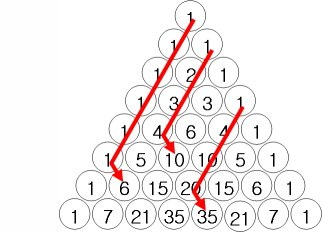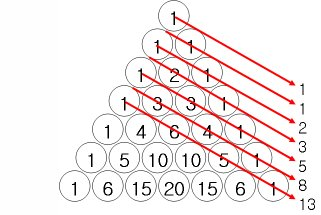# Pascal’s Triangle

Pascal's triangle is a triangular array of the binomial coefficients. each number is the sum of the two numbers directly above it.
Pascal's triangle has many properties and contains many patterns of numbers.

• The sum of the elements of row n is equal to 2n.• It is equal to the sum of the top sequences.• The 1st line = only 1's.
The 2nd line = the natural numbers in order.
The 3rd line = the triangular numbers in order.
The 4th line = the tetrahedral numbers in order.• Fibonacci numbersRelated Post